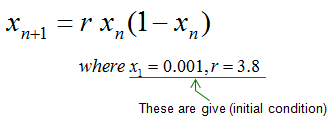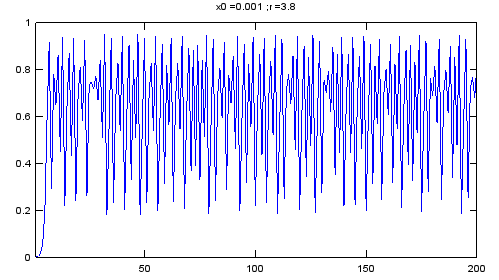Engineering Math - Quick Reference                                 Home : www.sharetechnote.com Recursive Function/Iterative Function   Recursion or Iteration means "doing the same process over and over".  If you represent this expression in mathematical form, it can be expressed as shown below. I hope this make sense to you.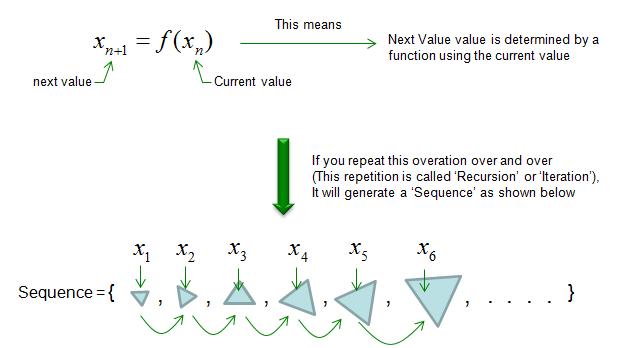If you illustrate the iterative/recursive process in more intuitive form, you can illustrate it as follows. If we take a function (f(x)) as a machine to generate a product(output) from a raw material (input). In this machine, if you put anything (input = raw material), you would get a product (output). And then you take the output and put it as the input of the machine, you would get another output. And then you take that output and put it as an input. you would get another output. This kind of repeating process is called 'iterative or recursive' process.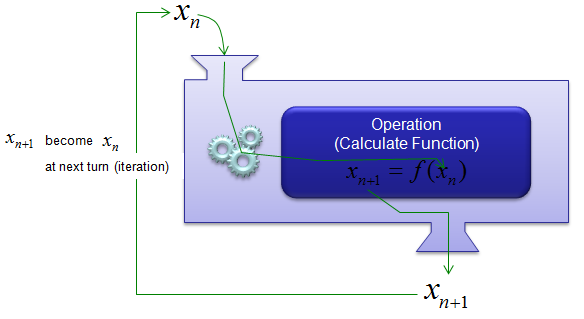Why we care about this kind of process ? One of the main reason is that the output of even a very simple iterative function generate very complex-looking output. In many cases, when we meet a very complicated things (phenomena), we tend to day "This is too complicated to explain in a certain scientific rule" and give up trying to find rule itself. For example, what would you say if somebody ask you "Look at all the branches of that big tree. Would that be any rules how each of those branches are formed ?. In other words, can you draw anything like those tree using some mathematical procedure ?". or what would you say if you are asked "Look at the spiky rocks, can you draw any similar shap like that using a mathematical procedure ?". Even until very recently (before we pay attention to 'iterative/recursive' functions), the questions to these questions were ".. It is just natural phenomena.. there is no specific rules .. or the rule is so complicated that we cannot explain in curent scientific method. But now a lot of those complicated phonomina can be explained by sprisingly simple iterative function or iterative process. and the iterative function/process is the foundation of Fractal and Chaos.     Example 1 >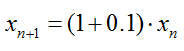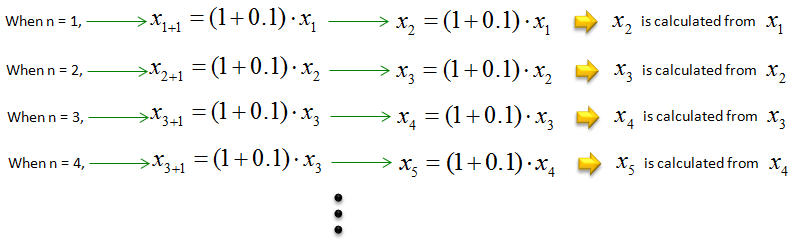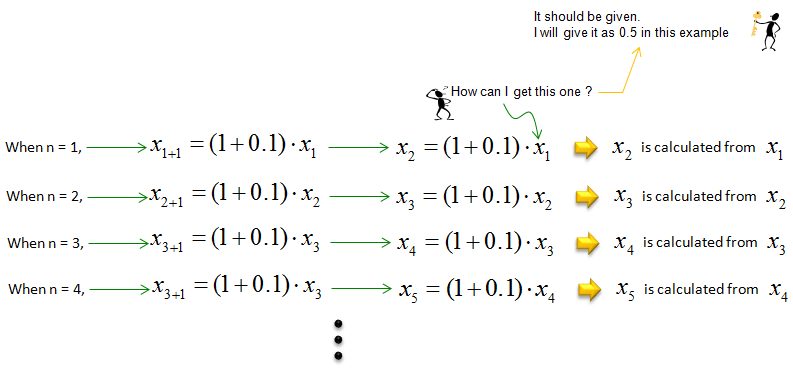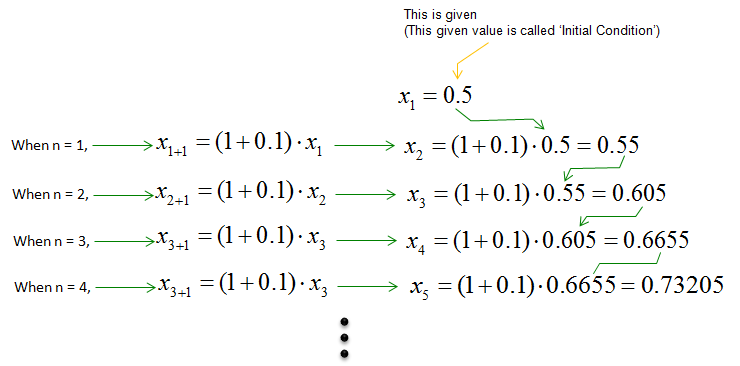Example 2 >